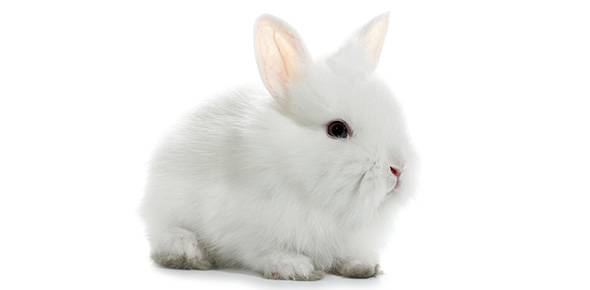Vestal Regular Jack Rabbit

4 Questions | Total Attempts: 107Settings• 1.
This picture represents a simplified version of the Jack Rabbit.  Which point shows the maximum kinetic energy?
• A.

A

• B.

B

• C.

C

• D.

H

• 2.
This picture represents a simplified version of the Jack Rabbit.  Choose the sentence that best describes what is happening between Point A and Point B.
• A.

The potential energy is decreasing.

• B.

The kinetic energy is decreasing.

• C.

The kinetic energy is increasing as the mechanical energy is increasing.

• D.

The potential energy is increasing as the kinetic energy is decreasing.

• 3.
On this ride, a roller coaster cart has a mass of approximately 4300 kg when full. The first vertical drop is 24.4 m. What is the velocity of the cart at the bottom of the first hill, if we neglect friction?
• A.

15.8 m/s

• B.

102 m/s

• C.

66.5 m/s

• D.

21.9 m/s

• 4.
On this ride, a roller coaster cart has a mass of approximately 4300 kg when full. The first vertical drop is 24.4 m. The next hill is 12.2 m tall. What is the velocity of the cart at the top of the second hill, if we neglect friction?
• A.

15.3 m/s

• B.

102 m/s

• C.

66.5 m/s

• D.

21.9 m/s

Related TopicsBack to top